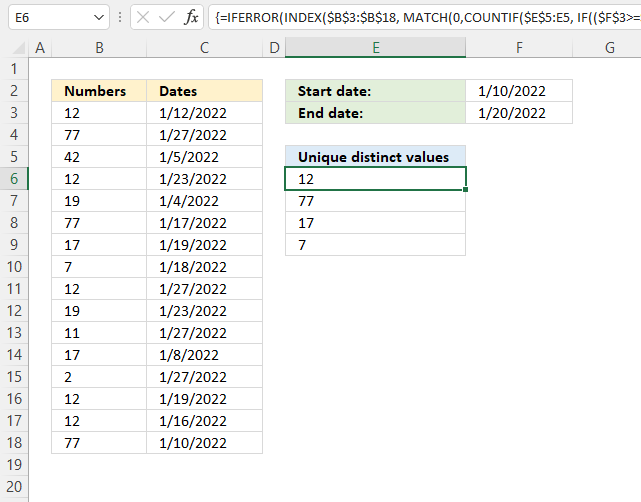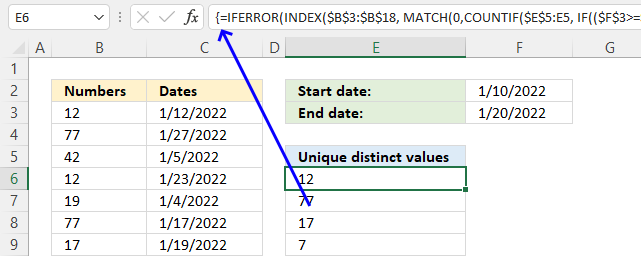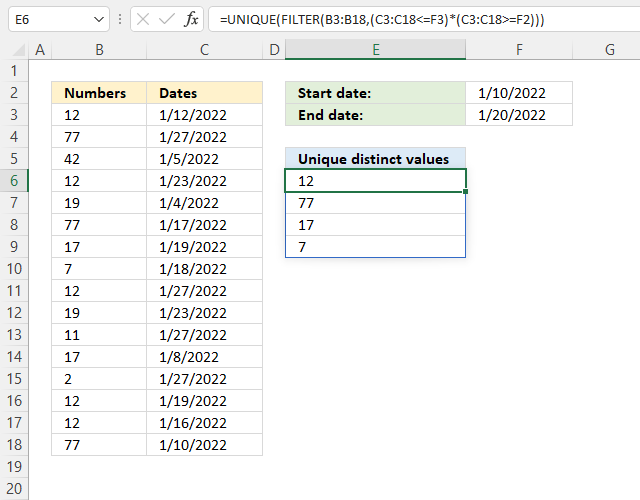Author: Oscar Cronquist Article last updated on December 08, 2021Question: I have a large list of dates and other adjacent values. I want to create a distinct list from the large list based on criteria, only values within a specific date interval.

## 1. Filter unique distinct values based on a date rangeThe image above shows a formula in cell E6 that extracts unique distinct values if the corresponding dates match the date range specified in cells F2 and F3.

The date range is specified in cell G1 and G2, see image above.

Array formula in cell D2:

=IFERROR(INDEX(\$B\$3:\$B\$18, MATCH(0,COUNTIF(\$E\$5:E5, IF((\$F\$3>=\$C\$3:\$C\$18)*(\$F\$2<=\$C\$3:\$C\$18), \$B\$3:\$B\$18, \$E\$5)), 0)), "")

### 1.1 How to enter an array formula

To enter an array formula, type the formula in a cell then press and hold CTRL + SHIFT simultaneously, now press Enter once. Release all keys.

The formula bar now shows the formula with a beginning and ending curly bracket telling you that you entered the formula successfully. Don't enter the curly brackets yourself.### 1.2 Explaining the formula in cell D2

#### Step 1 - Which dates are later than or equal to the start date?

The less than sign and equal sign are logical operators, they allow you to compare numbers and text strings. The result are boolean values and is either TRUE or FALSE.

\$F\$3>=\$C\$3:\$C\$18

becomes

44581>={44573; 44588; 44566; 44584; 44565; 44578; 44580; 44579; 44588; 44584; 44588; 44569; 44588; 44580; 44577; 44571}

and returns

{TRUE; FALSE; TRUE; FALSE; TRUE; TRUE; TRUE; TRUE; FALSE; FALSE; FALSE; TRUE; FALSE; TRUE; TRUE; TRUE}.

#### Step 2 - Which dates are earlier than or equal to the end date?

\$F\$2<=\$C\$3:\$C\$18

becomes

44571<={44573; 44588; 44566; 44584; 44565; 44578; 44580; 44579; 44588; 44584; 44588; 44569; 44588; 44580; 44577; 44571}

and returns

{TRUE; TRUE; FALSE; TRUE; FALSE; TRUE; TRUE; TRUE; TRUE; TRUE; TRUE; FALSE; TRUE; TRUE; TRUE; TRUE}.

#### Step 3 - AND logic

We need to identify dates that meet both conditions, we can do that by multiplying the arrays. The parentheses allow us to control the order of operation.

(\$F\$3>=\$C\$3:\$C\$18)*(\$F\$2<=\$C\$3:\$C\$18)

becomes

{TRUE; FALSE; TRUE; FALSE; TRUE; TRUE; TRUE; TRUE; FALSE; FALSE; FALSE; TRUE; FALSE; TRUE; TRUE; TRUE}*{TRUE; TRUE; FALSE; TRUE; FALSE; TRUE; TRUE; TRUE; TRUE; TRUE; TRUE; FALSE; TRUE; TRUE; TRUE; TRUE}

and returns

{1; 0; 0; 0; 0; 1; 1; 1; 0; 0; 0; 0; 0; 1; 1; 1}.

#### Step 4 - Replace 1 with the corresponding number on the same row

The IF function returns one value if the logical test is TRUE and another value if the logical test is FALSE.

IF(logical_test, [value_if_true], [value_if_false])

IF((\$F\$3>=\$C\$3:\$C\$18)*(\$F\$2<=\$C\$3:\$C\$18), \$B\$3:\$B\$18, \$E\$5)

becomes

IF({1; 0; 0; 0; 0; 1; 1; 1; 0; 0; 0; 0; 0; 1; 1; 1}, \$B\$3:\$B\$18, \$E\$5)

becomes

IF({1; 0; 0; 0; 0; 1; 1; 1; 0; 0; 0; 0; 0; 1; 1; 1}, {12; 77; 42; 12; 19; 77; 17; 7; 12; 19; 11; 17; 2; 12; 12; 77}, "Unique distinct values")

and returns

{12; "Unique distinct values"; "Unique distinct values"; "Unique distinct values"; "Unique distinct values"; 77; 17; 7; "Unique distinct values"; "Unique distinct values"; "Unique distinct values"; "Unique distinct values"; "Unique distinct values"; 12; 12; 77}.

#### Step 5 - Count previous displayed values

The COUNTIF function calculates the number of cells that is equal to a condition.

COUNTIF(rangecriteria)

COUNTIF(\$E\$5:E5, IF((\$F\$3>=\$C\$3:\$C\$18)*(\$F\$2<=\$C\$3:\$C\$18), \$B\$3:\$B\$18, \$E\$5))

becomes

COUNTIF(\$E\$5:E5, {12; "Unique distinct values"; "Unique distinct values"; "Unique distinct values"; "Unique distinct values"; 77; 17; 7; "Unique distinct values"; "Unique distinct values"; "Unique distinct values"; "Unique distinct values"; "Unique distinct values"; 12; 12; 77})

becomes

COUNTIF("Unique distinct values", {12; "Unique distinct values"; "Unique distinct values"; "Unique distinct values"; "Unique distinct values"; 77; 17; 7; "Unique distinct values"; "Unique distinct values"; "Unique distinct values"; "Unique distinct values"; "Unique distinct values"; 12; 12; 77})

and returns

{0; 1; 1; 1; 1; 0; 0; 0; 1; 1; 1; 1; 1; 0; 0; 0}.

#### Step 6 - Find first not displayed values in array

The MATCH function returns the relative position of an item in an array or cell reference that matches a specified value in a specific order.

MATCH(lookup_value, lookup_array, [match_type])

MATCH(0,COUNTIF(\$E\$5:E5, IF((\$F\$3>=\$C\$3:\$C\$18)*(\$F\$2<=\$C\$3:\$C\$18), \$B\$3:\$B\$18, \$E\$5)), 0)

becomes

MATCH(0,{0; 1; 1; 1; 1; 0; 0; 0; 1; 1; 1; 1; 1; 0; 0; 0}, 0)

and returns 1.

#### Step 7 - Get value

The INDEX function returns a value from a cell range, you specify which value based on a row and column number.

INDEX(array[row_num][column_num], [area_num])

INDEX(\$B\$3:\$B\$18, MATCH(0,COUNTIF(\$E\$5:E5, IF((\$F\$3>=\$C\$3:\$C\$18)*(\$F\$2<=\$C\$3:\$C\$18), \$B\$3:\$B\$18, \$E\$5)), 0))

becomes

INDEX(\$B\$3:\$B\$18, 1)

and returns 12 in cell E6.

#### Step 8 - Remove error values

The IFERROR function handles error values which the formula returns when no more values can be displayed.

IFERROR(INDEX(\$B\$3:\$B\$18, MATCH(0,COUNTIF(\$E\$5:E5, IF((\$F\$3>=\$C\$3:\$C\$18)*(\$F\$2<=\$C\$3:\$C\$18), \$B\$3:\$B\$18, \$E\$5)), 0)), "")

## 2. Filter unique distinct values based on a date range (Excel 365)Dynamic array formula in cell E6:

=UNIQUE(FILTER(B3:B18, (C3:C18<=F3)*(C3:C18>=F2)))

### 2.1 How to enter a dynamic array formula

The dynamic array formula is a new feature in Excel 365, you enter it as a regular formula.

### 2.2 Explaining dynamic array formula

#### Step 1 - Find dates earlier than or equal to end date

The less than sign and equal sign are logical operators, they allow you to compare numbers and text strings. The result is boolean values and the result is either TRUE or FALSE.

C3:C18<=F3

becomes

{44573; 44588; 44566; 44584; 44565; 44578; 44580; 44579; 44588; 44584; 44588; 44569; 44588; 44580; 44577; 44571}<=44581

and returns

{TRUE; FALSE; TRUE; FALSE; TRUE; TRUE; TRUE; TRUE; FALSE; FALSE; FALSE; TRUE; FALSE; TRUE; TRUE; TRUE}

#### Step 2 - Find dates later than or equal to start date

C3:C18>=F2

becomes

{44573; 44588; 44566; 44584; 44565; 44578; 44580; 44579; 44588; 44584; 44588; 44569; 44588; 44580; 44577; 44571}>=44571

and returns

{TRUE; TRUE; FALSE; TRUE; FALSE; TRUE; TRUE; TRUE; TRUE; TRUE; TRUE; FALSE; TRUE; TRUE; TRUE; TRUE}.

#### Step 3 - AND logic

Both tests must evaluate to true and to do that we need to multiply the array, in other words, apply AND logic.

Use the asterisk to multiply values or arrays.

* (asterisk) - Both logical expressions must match (AND logic)

The AND logic behind this is that

TRUE * TRUE = TRUE (1)
TRUE * FALSE = FALSE (0)
FALSE * FALSE = FALSE (0)

The parentheses control the order of operation.

(C3:C18<=F3)*(C3:C18>=F2)

becomes

{TRUE; FALSE; TRUE; FALSE; TRUE; TRUE; TRUE; TRUE; FALSE; FALSE; FALSE; TRUE; FALSE; TRUE; TRUE; TRUE}*{TRUE; TRUE; FALSE; TRUE; FALSE; TRUE; TRUE; TRUE; TRUE; TRUE; TRUE; FALSE; TRUE; TRUE; TRUE; TRUE}

and returns

{1; 0; 0; 0; 0; 1; 1; 1; 0; 0; 0; 0; 0; 1; 1; 1}.

#### Step 4 - Filter values based on conditions

The FILTER function lets you extract values/rows based on a condition or criteria. It is in the Lookup and reference category and is only available to Excel 365 subscribers.

FILTER(array, include, [if_empty])

FILTER(B3:B18, (C3:C18<=F3)*(C3:C18>=F2))

becomes

FILTER(B3:B18, {1; 0; 0; 0; 0; 1; 1; 1; 0; 0; 0; 0; 0; 1; 1; 1})

and returns

{12; 77; 17; 7; 12; 12; 77}.

#### Step 5 - Extract unique distinct values based on array

The UNIQUE function lets you extract both unique and unique distinct values and also compare columns to columns or rows to rows.

UNIQUE(array,[by_col],[exactly_once])

UNIQUE(FILTER(B3:B18, (C3:C18<=F3)*(C3:C18>=F2)))

becomes

UNIQUE({12; 77; 17; 7; 12; 12; 77})

and returns {12; 77; 17; 7}.